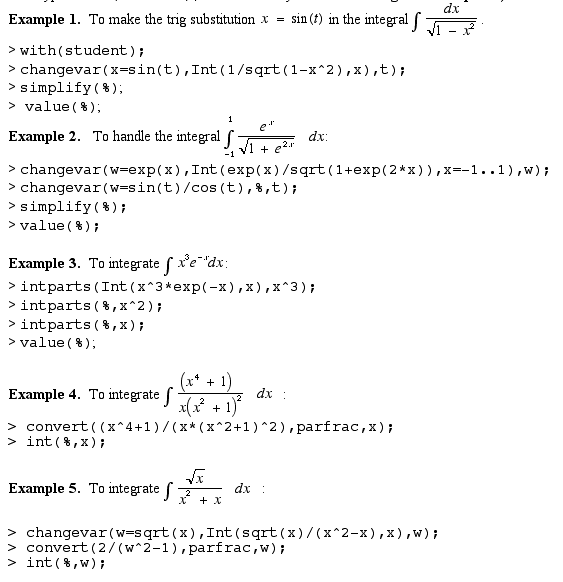### Code for the procedure "compareValues"

(copy appears between bars and paste into Maple)

```
compareValues := proc(f,a,b,n) local
A,B,c,d,i,j;

A:=array(1..6);
B:=array(1..6);

A:=`    Left Endpoint Rule:  `;
A:=`    Right Endpoint Rule: `;
A:=`    Midpoint Rule:       `;
A:=`    Trapezoid Rule:      `;
A:=`    Simpson's Rule:      `;
A:=`    Actual Value:        `;
B:= evalf(leftsum(f,x=a..b,n));
B:= evalf(rightsum(f,x=a..b,n));
B:= evalf(middlesum(f,x=a..b,n));
B:= evalf(trapezoid(f,x=a..b,n));
B:= evalf(simpson(f,x=a..b,n));
B:= evalf(int(f,x=a..b));

for i from 5 by -1 to 1 do
for j from 1 to i do
if(B[j]>B[j+1]) then
c:=B[j];
B[j]:=B[j+1];
B[j+1]:=c;
d:=A[j];
A[j]:=A[j+1];
A[j+1]:=d;
fi;
od;
od;

for i by 1 to 6 do
lprint(A[i],B[i])
od;
end;
```

### Review Examples